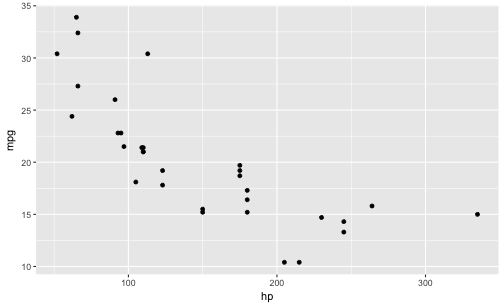# Covariance as correlation

Correlation is one of the most widely used and a well-known measure of the assocation (linear association, that is) of two variables.

Perhaps less well-known is that the correlation is in principle analoguous to the covariation.

To see this, consider the a formula of the covariance of two empirical datasets, $X$ and $Y$:

$$COV(X,Y) = \frac{1}{n} \cdot \big( \sum (X_i -\bar{X}) \cdot (Y_i - \bar{Y}) \big)$$

In other words, the covariance of $X$ and $Y$ $COV(X,Y)$ is the average of difference of some value to its mean.

This idea is conveyed by this picture:# The covariance is identical to the correlation (?)

What does it mean to say the (coefficient of) correlation is “identical” to the covariation?

If we “feed” z-scaled values to the covariation, we will get back the correlation.

In other words, the correlation equals the covariation if the data are z-scaled.

So, let’s see. We replace $X$ by $z_X$ and $Y$ by $z_Y$ and see what happens.

$$Cov(z_X, z_Y) = \frac{1}{n} \cdot \sum$$

\sum (z_{X_i} - z_{\bar{X}}) (z_{Y_i} - z_{\bar{Y}})

However, $z_{\bar{X}} = 0$, and by analogy, $z_{\bar{y}} = 0$. So the eqaution simplifies to

$$Cov(z_X, z_Y) = \frac{1}{n} \cdot \sum (z_{X_i} \cdot z_{Y_i})$$

Now, $z_x$ can be expressed as

$$z_x = \frac{X_i - \bar{X}}{sd_X}$$

The same rule applies for $z_y$ by analogy.

Now, let’s insert the previous equation in the equation of $Cov(z_X, z_Y)$:

$$Cov(z_X, z_Y) = \frac{1}{n} \cdot \big( \sum \frac{X_i - \bar{X}}{sd_X} \cdot \frac{Y_i - \bar{Y}}{sd_Y} \big)$$

$sd_X$ and $sd_Y$ can be pulled out of the sum, right at the front of the equation, leaving us with

$$Cov(z_X, z_Y) = \frac{1}{sd_X \cdot sd_Y} \cdot \big( \sum (X_i - \bar{X}) \cdot (Y_i - \bar{Y}) \big)$$

And that’s the definition of the correlation of $X$ and $Y$, more frequently put this way:

$$Cov(z_X, z_Y) = \frac {\sum (X_i - \bar{X}) \cdot (Y_i - \bar{Y})}{sd_X \cdot sd_Y}$$

Hence,

$$Cov(z_X, z_Y) = cor(X,Y)$$

# Example time

It is helpful to consider an example.

This is a scatterplot of two variables, ie., “raw data” as is “fed in” for the calculation of the (empirical) covariation:

library(tidyverse)
mtcars %>%
ggplot +
aes(x = hp, y = mpg) +
geom_point()And now, let’s z-scale the two variables and draw the same diagram again:

mtcars %>%
select(hp, mpg) %>%
mutate_all(funs(scale)) %>%
ggplot +
aes(x = hp, y = mpg) +
geom_point()Now, what’s the difference? Nada, no difference. That’s reassuring, because we just derived that the assocation of the variables is the same - no matter if use the raw data or z-scaled data as input. The diagrams confirms this in an more intuitive way.

# Summary

The correlation is a “special case” of the covariance; it is the case when we feed z-scaled data to the covariance.

Happy data analyzing!